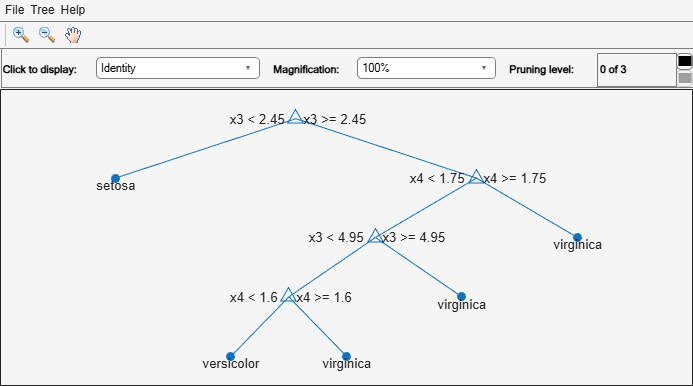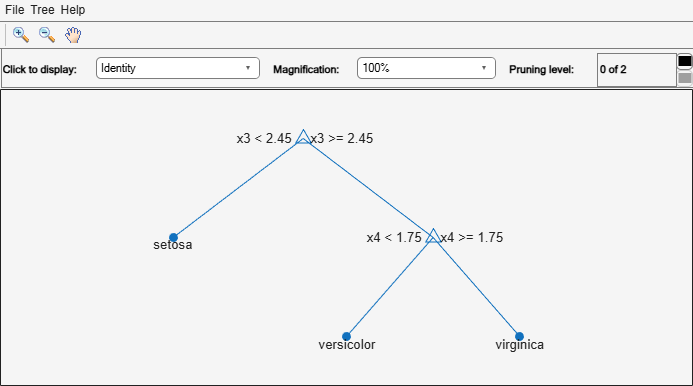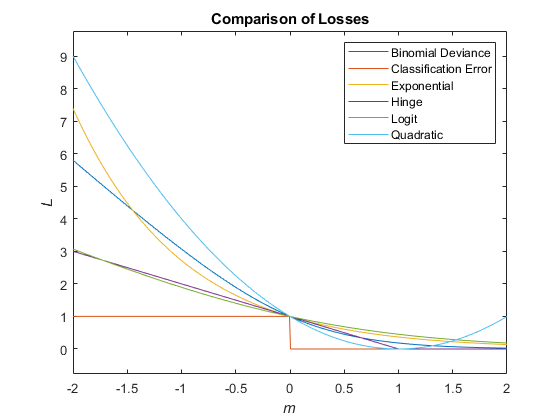# loss

Classification error

## Syntax

``L = loss(tree,TBL,ResponseVarName)``
``L = loss(tree,TBL,Y)``
``L = loss(tree,X,Y)``
``L = loss(___,Name=Value)``
``````[L,se,NLeaf,bestlevel] = loss(___)``````

## Description

````L = loss(tree,TBL,ResponseVarName)` returns a scalar representing how well `tree` classifies the data in `TBL`, when `TBL.ResponseVarName` contains the true classifications.When computing the loss, `loss` normalizes the class probabilities in `Y` to the class probabilities used for training, stored in the `Prior` property of `tree`.```
````L = loss(tree,TBL,Y)` returns a scalar representing how well `tree` classifies the data in `TBL`, when `Y` contains the true classifications.```

example

````L = loss(tree,X,Y)` returns a scalar representing how well `tree` classifies the data in `X`, when `Y` contains the true classifications.```

example

````L = loss(___,Name=Value)` returns the loss with additional options specified by one or more name-value arguments, using any of the previous syntaxes. For example, you can specify the loss function or observation weights.```
``````[L,se,NLeaf,bestlevel] = loss(___)``` also returns the vector of standard errors of the classification errors (`se`), the vector of numbers of leaf nodes (`NLeaf`) in the trees of the pruning sequence, and the best pruning level (`bestlevel`) as defined in the `TreeSize` name-value argument.```

## Examples

collapse all

Compute the resubstituted classification error for the `ionosphere` data set.

```load ionosphere tree = fitctree(X,Y); L = loss(tree,X,Y)```
```L = 0.0114 ```

Unpruned decision trees tend to overfit. One way to balance model complexity and out-of-sample performance is to prune a tree (or restrict its growth) so that in-sample and out-of-sample performance are satisfactory.

Load Fisher's iris data set. Partition the data into training (50%) and validation (50%) sets.

```load fisheriris n = size(meas,1); rng(1) % For reproducibility idxTrn = false(n,1); idxTrn(randsample(n,round(0.5*n))) = true; % Training set logical indices idxVal = idxTrn == false; % Validation set logical indices```

Grow a classification tree using the training set.

`Mdl = fitctree(meas(idxTrn,:),species(idxTrn));`

View the classification tree.

`view(Mdl,'Mode','graph');`The classification tree has four pruning levels. Level 0 is the full, unpruned tree (as displayed). Level 3 is just the root node (i.e., no splits).

Examine the training sample classification error for each subtree (or pruning level) excluding the highest level.

```m = max(Mdl.PruneList) - 1; trnLoss = resubLoss(Mdl,'SubTrees',0:m)```
```trnLoss = 3×1 0.0267 0.0533 0.3067 ```
• The full, unpruned tree misclassifies about 2.7% of the training observations.

• The tree pruned to level 1 misclassifies about 5.3% of the training observations.

• The tree pruned to level 2 (i.e., a stump) misclassifies about 30.6% of the training observations.

Examine the validation sample classification error at each level excluding the highest level.

`valLoss = loss(Mdl,meas(idxVal,:),species(idxVal),'SubTrees',0:m)`
```valLoss = 3×1 0.0369 0.0237 0.3067 ```
• The full, unpruned tree misclassifies about 3.7% of the validation observations.

• The tree pruned to level 1 misclassifies about 2.4% of the validation observations.

• The tree pruned to level 2 (i.e., a stump) misclassifies about 30.7% of the validation observations.

To balance model complexity and out-of-sample performance, consider pruning `Mdl` to level 1.

```pruneMdl = prune(Mdl,'Level',1); view(pruneMdl,'Mode','graph')```## Input Arguments

collapse all

Trained classification tree, specified as a `ClassificationTree` or `CompactClassificationTree` model object. That is, `tree` is a trained classification model returned by `fitctree` or `compact`.

Sample data, specified as a table. Each row of `TBL` corresponds to one observation, and each column corresponds to one predictor variable. Optionally, `TBL` can contain additional columns for the response variable and observation weights. `TBL` must contain all the predictors used to train `tree`. Multicolumn variables and cell arrays other than cell arrays of character vectors are not allowed.

If `TBL` contains the response variable used to train `tree`, then you do not need to specify `ResponseVarName` or `Y`.

If you train `tree` using sample data contained in a `table`, then the input data for this method must also be in a table.

Data Types: `table`

Data to classify, specified as a numeric matrix. Each row of `X` represents one observation, and each column represents one predictor. `X` must have the same number of columns as the data used to train `tree`. `X` must have the same number of rows as the number of elements in `Y`.

Data Types: `single` | `double`

Response variable name, specified as the name of a variable in `TBL`. If `TBL` contains the response variable used to train `tree`, then you do not need to specify `ResponseVarName`.

If you specify `ResponseVarName`, then you must do so as a character vector or string scalar. For example, if the response variable is stored as `TBL.Response`, then specify it as `'Response'`. Otherwise, the software treats all columns of `TBL`, including `TBL.ResponseVarName`, as predictors.

The response variable must be a categorical, character, or string array, logical or numeric vector, or cell array of character vectors. If the response variable is a character array, then each element must correspond to one row of the array.

Data Types: `char` | `string`

Class labels, specified as a categorical, character, or string array, a logical or numeric vector, or a cell array of character vectors. `Y` must be of the same type as the classification used to train `tree`, and its number of elements must equal the number of rows of `X`.

Data Types: `categorical` | `char` | `string` | `logical` | `single` | `double` | `cell`

### Name-Value Arguments

Specify optional pairs of arguments as `Name1=Value1,...,NameN=ValueN`, where `Name` is the argument name and `Value` is the corresponding value. Name-value arguments must appear after other arguments, but the order of the pairs does not matter.

Example: `L = loss(tree,X,Y,LossFun="exponential")` specifies an exponential loss function

Before R2021a, use commas to separate each name and value, and enclose `Name` in quotes.

Loss Function and Weights

collapse all

Loss function, specified as a built-in loss function name or function handle.

• This table lists the built-in loss function names. Specify a loss function using its corresponding character vector or string scalar.

ValueDescription
`"binodeviance"`Binomial deviance
`"classifcost"`Observed misclassification cost
`"classiferror"`Misclassified rate in decimal
`"exponential"`Exponential loss
`"hinge"`Hinge loss
`"logit"`Logistic loss
`"mincost"`Minimal expected misclassification cost (for classification scores that are posterior probabilities)
`"quadratic"`Quadratic loss

`"mincost"` is appropriate for classification scores that are posterior probabilities. Classification trees return posterior probabilities as classification scores by default (see `predict`).

• Specify your own function using function handle notation.

Suppose that `n` be the number of observations in `X` and `K` be the number of distinct classes (`numel(tree.ClassNames)`). Your function must have this signature

``lossvalue = lossfun(C,S,W,Cost)``
where:

• The output argument `lossvalue` is a scalar.

• You choose the function name (`lossfun`).

• `C` is an `n`-by-`K` logical matrix with rows indicating which class the corresponding observation belongs. The column order corresponds to the class order in `tree.ClassNames`.

Construct `C` by setting `C(p,q) = 1` if observation `p` is in class `q`, for each row. Set all other elements of row `p` to `0`.

• `S` is an `n`-by-`K` numeric matrix of classification scores. The column order corresponds to the class order in `tree.ClassNames`. `S` is a matrix of classification scores, similar to the output of `predict`.

• `W` is an `n`-by-1 numeric vector of observation weights. If you pass `W`, the software normalizes them to sum to `1`.

• `Cost` is a K-by-`K` numeric matrix of misclassification costs. For example, ```Cost = ones(K) - eye(K)``` specifies a cost of `0` for correct classification, and `1` for misclassification.

Specify your function using `LossFun=@lossfun`.

For more details on loss functions, see Classification Loss.

Data Types: `char` | `string` | `function_handle`

Observation weights, specified as a numeric vector of positive values or the name of a variable in `TBL`.

If you specify `Weights` as a numeric vector, then the size of `Weights` must be equal to the number of rows in `X` or `TBL`.

If you specify `Weights` as the name of a variable in `TBL`, you must do so as a character vector or string scalar. For example, if the weights are stored as `TBL.W`, then specify `Weights` as `"W"`. Otherwise, the software treats all columns of `TBL`, including `TBL.W`, as predictors.

`loss` normalizes the weights so that observation weights in each class sum to the prior probability of that class. When you specify `Weights`, `loss` computes weighted classification loss.

Data Types: `single` | `double` | `char` | `string`

Options for Pruning Subtrees

collapse all

Pruning level, specified as a vector of nonnegative integers in ascending order or `"all"`.

If you specify a vector, then all elements must be at least `0` and at most `max(tree.PruneList)`. `0` indicates the full, unpruned tree and `max(tree.PruneList)` indicates the completely pruned tree (i.e., just the root node).

If you specify `"all"`, then `loss` operates on all subtrees (in other words, the entire pruning sequence). This specification is equivalent to using `0:max(tree.PruneList)`.

`loss` prunes `tree` to each level indicated in `Subtrees`, and then estimates the corresponding output arguments. The size of `Subtrees` determines the size of some output arguments.

To invoke `Subtrees`, the properties `PruneList` and `PruneAlpha` of `tree` must be nonempty. In other words, grow `tree` by setting `Prune="on"`, or by pruning `tree` using `prune`.

Example: `Subtrees="all"`

Data Types: `single` | `double` | `char` | `string`

Tree size, specified as one of these values:

• `"se"``loss` returns the highest pruning level with loss within one standard deviation of the minimum (`L`+`se`, where `L` and `se` relate to the smallest value in `Subtrees`).

• `"min"``loss` returns the element of `Subtrees` with smallest loss, usually the smallest element of `Subtrees`.

## Output Arguments

collapse all

Classification loss, returned as a vector the length of `Subtrees`. The meaning of the error depends on the values in `Weights` and `LossFun`.

Standard error of loss, returned as a vector the length of `Subtrees`.

Number of leaves (terminal nodes) in the pruned subtrees, returned as a vector the length of `Subtrees`.

Best pruning level as defined in the `TreeSize` name-value argument, returned as a scalar whose value depends on `TreeSize`:

• `TreeSize` = `"se"``loss` returns the highest pruning level with loss within one standard deviation of the minimum (`L`+`se`, where `L` and `se` relate to the smallest value in `Subtrees`).

• `TreeSize` = `"min"``loss` returns the element of `Subtrees` with smallest loss, usually the smallest element of `Subtrees`.

By default, `bestlevel` is the pruning level that gives loss within one standard deviation of minimal loss.

collapse all

### Classification Loss

Classification loss functions measure the predictive inaccuracy of classification models. When you compare the same type of loss among many models, a lower loss indicates a better predictive model.

Consider the following scenario.

• L is the weighted average classification loss.

• n is the sample size.

• For binary classification:

• yj is the observed class label. The software codes it as –1 or 1, indicating the negative or positive class (or the first or second class in the `ClassNames` property), respectively.

• f(Xj) is the positive-class classification score for observation (row) j of the predictor data X.

• mj = yjf(Xj) is the classification score for classifying observation j into the class corresponding to yj. Positive values of mj indicate correct classification and do not contribute much to the average loss. Negative values of mj indicate incorrect classification and contribute significantly to the average loss.

• For algorithms that support multiclass classification (that is, K ≥ 3):

• yj* is a vector of K – 1 zeros, with 1 in the position corresponding to the true, observed class yj. For example, if the true class of the second observation is the third class and K = 4, then y2* = [`0 0 1 0`]′. The order of the classes corresponds to the order in the `ClassNames` property of the input model.

• f(Xj) is the length K vector of class scores for observation j of the predictor data X. The order of the scores corresponds to the order of the classes in the `ClassNames` property of the input model.

• mj = yj*f(Xj). Therefore, mj is the scalar classification score that the model predicts for the true, observed class.

• The weight for observation j is wj. The software normalizes the observation weights so that they sum to the corresponding prior class probability stored in the `Prior` property. Therefore,

`$\sum _{j=1}^{n}{w}_{j}=1.$`

Given this scenario, the following table describes the supported loss functions that you can specify by using the `LossFun` name-value argument.

Loss FunctionValue of `LossFun`Equation
Binomial deviance`"binodeviance"`$L=\sum _{j=1}^{n}{w}_{j}\mathrm{log}\left\{1+\mathrm{exp}\left[-2{m}_{j}\right]\right\}.$
Observed misclassification cost`"classifcost"`

$L=\sum _{j=1}^{n}{w}_{j}{c}_{{y}_{j}{\stackrel{^}{y}}_{j}},$

where ${\stackrel{^}{y}}_{j}$ is the class label corresponding to the class with the maximal score, and ${c}_{{y}_{j}{\stackrel{^}{y}}_{j}}$ is the user-specified cost of classifying an observation into class ${\stackrel{^}{y}}_{j}$ when its true class is yj.

Misclassified rate in decimal`"classiferror"`

$L=\sum _{j=1}^{n}{w}_{j}I\left\{{\stackrel{^}{y}}_{j}\ne {y}_{j}\right\},$

where I{·} is the indicator function.

Cross-entropy loss`"crossentropy"`

`"crossentropy"` is appropriate only for neural network models.

The weighted cross-entropy loss is

`$L=-\sum _{j=1}^{n}\frac{{\stackrel{˜}{w}}_{j}\mathrm{log}\left({m}_{j}\right)}{Kn},$`

where the weights ${\stackrel{˜}{w}}_{j}$ are normalized to sum to n instead of 1.

Exponential loss`"exponential"`$L=\sum _{j=1}^{n}{w}_{j}\mathrm{exp}\left(-{m}_{j}\right).$
Hinge loss`"hinge"`$L=\sum _{j=1}^{n}{w}_{j}\mathrm{max}\left\{0,1-{m}_{j}\right\}.$
Logit loss`"logit"`$L=\sum _{j=1}^{n}{w}_{j}\mathrm{log}\left(1+\mathrm{exp}\left(-{m}_{j}\right)\right).$
Minimal expected misclassification cost`"mincost"`

`"mincost"` is appropriate only if classification scores are posterior probabilities.

The software computes the weighted minimal expected classification cost using this procedure for observations j = 1,...,n.

1. Estimate the expected misclassification cost of classifying the observation Xj into the class k:

`${\gamma }_{jk}={\left(f{\left({X}_{j}\right)}^{\prime }C\right)}_{k}.$`

f(Xj) is the column vector of class posterior probabilities for the observation Xj. C is the cost matrix stored in the `Cost` property of the model.

2. For observation j, predict the class label corresponding to the minimal expected misclassification cost:

`${\stackrel{^}{y}}_{j}=\underset{k=1,...,K}{\text{argmin}}{\gamma }_{jk}.$`

3. Using C, identify the cost incurred (cj) for making the prediction.

The weighted average of the minimal expected misclassification cost loss is

`$L=\sum _{j=1}^{n}{w}_{j}{c}_{j}.$`

Quadratic loss`"quadratic"`$L=\sum _{j=1}^{n}{w}_{j}{\left(1-{m}_{j}\right)}^{2}.$

If you use the default cost matrix (whose element value is 0 for correct classification and 1 for incorrect classification), then the loss values for `"classifcost"`, `"classiferror"`, and `"mincost"` are identical. For a model with a nondefault cost matrix, the `"classifcost"` loss is equivalent to the `"mincost"` loss most of the time. These losses can be different if prediction into the class with maximal posterior probability is different from prediction into the class with minimal expected cost. Note that `"mincost"` is appropriate only if classification scores are posterior probabilities.

This figure compares the loss functions (except `"classifcost"`, `"crossentropy"`, and `"mincost"`) over the score m for one observation. Some functions are normalized to pass through the point (0,1).### True Misclassification Cost

The true misclassification cost is the cost of classifying an observation into an incorrect class.

You can set the true misclassification cost per class by using the `Cost` name-value argument when you create the classifier. `Cost(i,j)` is the cost of classifying an observation into class `j` when its true class is `i`. By default, `Cost(i,j)=1` if `i~=j`, and `Cost(i,j)=0` if `i=j`. In other words, the cost is `0` for correct classification and `1` for incorrect classification.

### Expected Misclassification Cost

The expected misclassification cost per observation is an averaged cost of classifying the observation into each class.

Suppose you have `Nobs` observations that you want to classify with a trained classifier, and you have `K` classes. You place the observations into a matrix `X` with one observation per row.

The expected cost matrix `CE` has size `Nobs`-by-`K`. Each row of `CE` contains the expected (average) cost of classifying the observation into each of the `K` classes. `CE(n,k)` is

`$\sum _{i=1}^{K}\stackrel{^}{P}\left(i|X\left(n\right)\right)C\left(k|i\right),$`

where:

• K is the number of classes.

• $\stackrel{^}{P}\left(i|X\left(n\right)\right)$ is the posterior probability of class i for observation X(n).

• $C\left(k|i\right)$ is the true misclassification cost of classifying an observation as k when its true class is i.

### Score (tree)

For trees, the score of a classification of a leaf node is the posterior probability of the classification at that node. The posterior probability of the classification at a node is the number of training sequences that lead to that node with the classification, divided by the number of training sequences that lead to that node.

For an example, see Posterior Probability Definition for Classification Tree.

## Version History

Introduced in R2011a